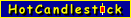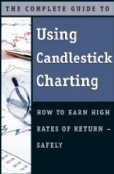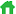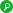Enter Symbol Lookup Patterns
Popular tickers today...

Daily ChartsAs Seen In...Who was Fibonacci ?Leonardo Pisano Bogollo (aka Fibonacci) (1170-1250), an Italian mathematician from Pisa is credited with introducing the Fibonacci sequence to Europe. The sequence is widely believed to originate from someone in India as early as the 6th century. Fibonacci also popularized the use of HinduArabic numerals (a replacement for Roman numerals) in Europe through his book Liber Abaci (Book of Abacus or Book of Calculation).

# What are Fibonacci Retracement Levels ?

Fibonacci retracement lines are well known in technical analysis of stocks and other securities. These horizontal lines represent potential support or resistance price levels. Common retracement values are 23.6%, 38.2% and 61.8% of the % price move within a trend. 50% is also used on the charts even though it is not a fibonacci retracement level based on the fibonacci sequence.

The fibonacci sequence starts with 0, 1 and continues to infinity. The sequence is written by taking the current number in the sequence and adding it to the previous number in the sequence. That result is the next number in the sequence. So we have...

0, 1 (0+1 = 1)
0, 1, 1 (1+1 = 2)
0, 1, 1, 2 (2+1 = 3)
0, 1, 1, 2, 3 (3+2 = 5)
0, 1, 1, 2, 3, 5 (5+3 = 8)
0, 1, 1, 2, 3, 5, 8 (8+5 = 13)
0, 1, 1, 2, 3, 5, 8, 13 (13+8 = 21)
0, 1, 1, 2, 3, 5, 8, 13, 21 (21+13 = 34)
0, 1, 1, 2, 3, 5, 8, 13, 21, 34 (34+21 = 55)
0, 1, 1, 2, 3, 5, 8, 13, 21, 34, 55 (55+34 = 89)
0, 1, 1, 2, 3, 5, 8, 13, 21, 34, 55, 89.... to infinity !

The fibonacci retracements are based on a unique property of this sequence.

Notice when you take any number in the sequence beyond the initial 0, 1 in the sequence and divide it by the next number you get an approximation to 0.618... (a fibonacci retracement level and inverse of the Golden Ratio). And, if you instead divide your number by 2 numbers ahead in the sequence you get an approximation to 0.382... (a fibonacci retracement level). And, if you instead divide your number by 3 numbers ahead in the sequence you get an approximation to 0.236... (a fibonacci retracement level). The accuracy of these approximations improves as the sequence extends to infinity.

Golden Ratio

A fundamental property of the fibonacci sequence is the Golden Ratio (1.6180339887...). The Golden Ratio is an irrational number. Take any number beyond the initial 0, 1, 1 in the sequence and divide it by the previous number and you get an approximation to 1.6180339887... that improves as the sequence continues.

The approximation to the Golden Ratio shows up frequently in nature.

1. Dividing the number female bees by the number of male bees in any given hive gives you approximately 1.618.

2. The count of the 2 opposing spiral patterns of seeds in sunflowers has a 1.618 ratio (commonly 89/55 or 55/34 or 34/21).

3. The DNA molecule, the program for all life, is based on the golden section. It measures 34 angstroms long by 21 angstroms wide for each full cycle of its double helix spiral. 34/21 = Golden Ratio.

4. Anatomy: Measure the length of your arm (from shoulder to tip of middle finger) then divide by the length from your elbow to the tip of your middle finger. The result will approximate 1.6180339887...

5. Other examples include atoms, pine cones, pineapples, nautilus shells, certain hurricanes and spiral galaxies.

You can also use the Golden Ratio to find any number in the fibonacci sequence. Here's how...

Let's say you want to find the 19th number in the fibonacci sequence. Let's call that variable y. You get your answer by raising the Golden Ratio to the 18th power and dividing by the square root of 5. Then round to the nearest integer. More generally it is ((Golden Ratio ^ (y-1)) / sqrt (5).

Use of Fibonacci Retracements

The price levels for fibonacci retracement lines depend on trend identification and determination of the high and low prices within the trend. Trend lines are subjective so caution should be used. Someone may draw a different trend line than the next person which in turn may change the price levels of the fibonacci retracement lines.

It is not recommended that you rely solely on Fibonacci retracements or HotCandlestick.com when making trading decisions. Candlestick patterns are nicely complimented by Fibonacci retracement levels. But you still need to do your own research.

HotCandlestick.com automatically determines the fibonacci retracement lines using the following method...

Identify clear price trends on the chart using the following criteria:

A) A trend must run over at least 10 consecutive (daily, weekly, monthly or quarterly) candlesticks.

B) A trend is nullified by too much price variation which can include a significant price gap.

A solid orange line is drawn on the chart for each of the last 2 up and down trends. For the most recent up trend, a black circle is drawn around the high and low. For the most recent down trend, a red circle is drawn around the high and low.

The fibonacci retracement price levels are calculated by removing 23.6%, 38.2%, 50% and 61.8% of the % price move.

The 4 fibonacci retracements are plotted as a series of horizontal dashed orange lines with the corresponding price levels shown on the chart. To avoid chart clutter this is done only for the most recent trend line.

Fibonacci retracements are automatically included on all daily, weekly, monthly and quarterly charts. Since prices are plotted on a logarithmic scale on the charts this means that any identical vertical distance on the chart is the same % price change. You can easily verify the retracement levels by holding a piece of paper to the chart and marking the distance from the circled high to the 50% retracement. Then measure the distance from the circled low to the 50% retracement. The 2 distances will be equal.

Next Page

» View the candlestick patterns tracked at HotCandlestick.com

Risk Statement